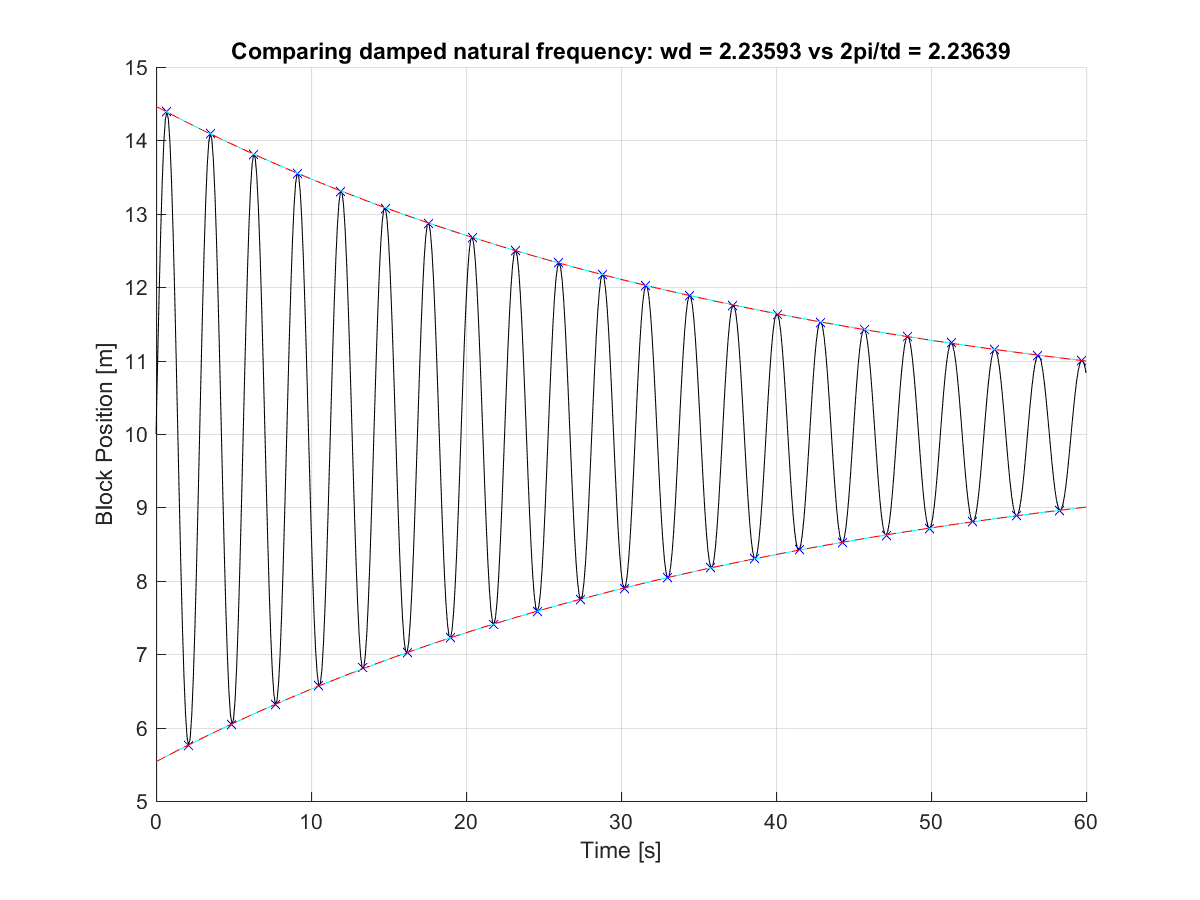# Ben's Website

Serious musings# Getting started in Simbody

I'm testing Simbody for mechanical system modeling, here are some quick notes.

# VisualStudio 2016 setup #

I followed the x64 VisualStudio instructions, placing the final installation in C:\Simbody. After creating a new C/C++ Win32 Console Application and a blank source file (to access the C/C++ options), I entered these project properties:  Configuration Properties | VC++ Directories | Executable Directories = C:\Simbody\bin   Configuration Properties | C/C++ | All Options | Additional Include Directories = C:\Simbody\include   Configuration Properties | Linker | All Options | Additional Library Directories = C:\Simbody\lib   Configuration Properties | Linker | All Options | Additional Dependencies = libblas.lib;liblapack.lib; pthreadVC2_x64.lib;SimTKcommon.lib;SimTKmath.lib; SimTKsimbody.lib (all in the lib directory)

I'm no expert on VS; the above work for me.

# A basic check of the dynamics #

Let's model the elementary mass/spring/damper system and calculate the natural frequency and damping from the response:See github and the many comments in verifyDynamics.cpp. Included there is a basic PositionReporter that writes positions into a given text file.

The values given in the figure allow calculation of the system's response, with some relevant parameters being:

$\omega_n = \sqrt{\frac{K_{spring}}{M}} = 2.2361$ [rad/s]

$\zeta = \frac{C_{damp}}{2 \sqrt{K_{spring}M}} = 0.0112$ (underdamped)

$\omega_d = \omega_n \sqrt{1-\zeta^2} = 2.2359$ [rad/s]

Now, let's measure these same parameters from the simulated response. Running verifyDyanmics.cpp produces a csv file with the x position written out every 0.1sec. Plotting this in Matlab gives:The response is the black line, with blue x's indicating the identified peaks and valleys.

The dashpot causes the initial 10m/s velocity to decay exponentially, with the response envelope given by $A e^{-\zeta \omega_n t}$. If we measure the time between peaks or valleys, we find the damped time constant $t_d$, which is related to the damped natural frequency by:

$\omega_d = \frac{2\pi}{t_d} = 2.3639$ versus $2.2359$ calculated above.

The above response also draws the envelope function, with the cyan giving the calculated envelope and the red dashed Matlab's 1D exponential estimate.

So, this was a very simple check on Simbody's physics and a good excuse to figure out how to easily move data out of Simbody.